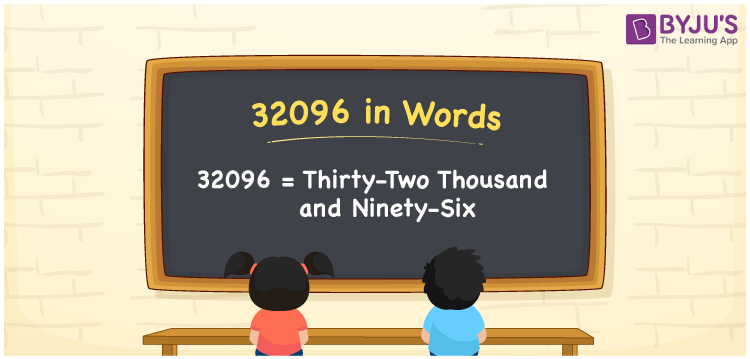32096 in words is written as thirty-two thousand and ninety-six. We can easily convert numbers into words using the place value system. For example, if you gave Rs. 32096 for charity, you can write it as “I have Rs. Thirty-two thousand and ninety-six for charity”. Also, 32096 is a cardinal number as it specifies the value.

 32096 in Words: Thirty-two Thousand and Ninety-six. Thirty-two Thousand and Ninety-six in Numerical Form: 32096.

## 32096 in English Words## How to Write 32096 in Words?

As the number 32096 has five digits, the place value table up to 5 digits is given below.

 Ten-thousands Thousands Hundreds Tens Ones 3 2 0 9 6

The expanded form of 32096 is as follows:

= 3 × Ten thousand + 2 × Thousand + 0 × Hundred + 9 × Ten + 6 × One

= 3 × 10000 + 2 × 1000 + 0 × 100 + 9 × 10 + 6 × 1

= 30000 + 2000 + 90 + 6

= 32096

= Thirty-two thousand and ninety-six

Hence, 32096 in words is thirty-two thousand and ninety-six.

32096 in words – Thirty-two thousand and ninety-six

Is 32096 an odd number? – No

Is 32096 an even number? – Yes

Is 32096 a perfect square number? – No

Is 32096 a perfect cube number? – No

Is 32096 a prime number? – No

Is 32096 a composite number? – Yes

## Frequently Asked Questions on 32096 in Words

Q1

### Write 32096 in words?

32096 in words is thirty-two thousand and ninety-six.

Q2

### Simplify 32000 + 96, and express it in words.

Simplifying 32000 + 96, we get 32096. Hence, 32096 in words is thirty-two thousand and ninety-six.

Q3

### Is 32096 a prime number?

No, 32096 is not a prime number.